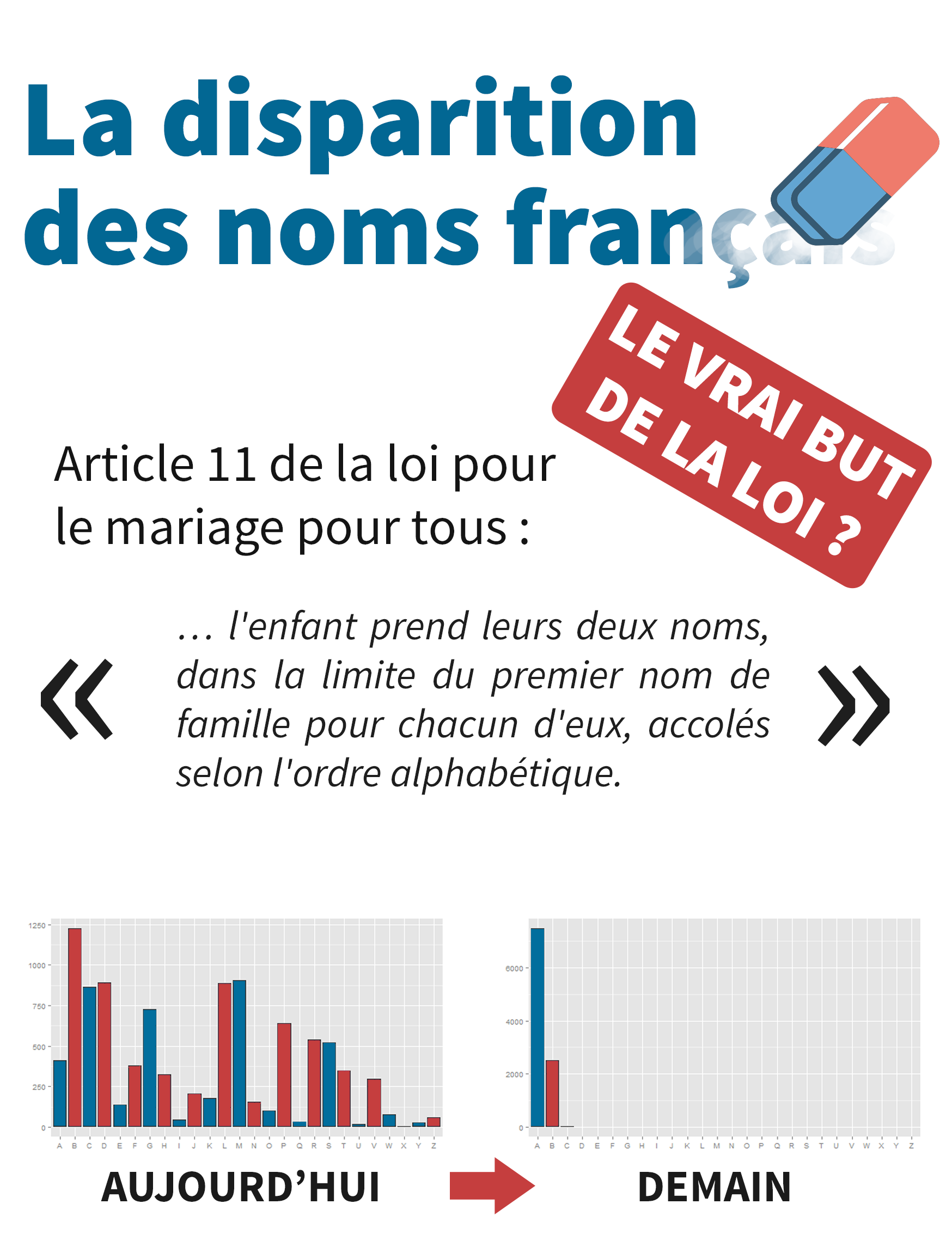## [Sampling] Why you should never ever use the word “representative” about survey sampling

We often hear the term “representative”, which supposedly assesses the quality of a sample. In this article, I’ll try to show you that not only “representative” has no scientific meaning, but also it is misleading, especially for people who are not survey sampling specialists. This includes students, researchers in social science who use surveys, journalists, etc. I hope I’ll convince you to never use it again !

I’ll try to make this post understandable even if you don’t know the basics of survey sampling theory. Well, you might miss a thing or two, but I think my main arguments are perfectly understandable to anyone who has ever taken a course in statistics. And perhaps I’ll write an article later to explain the basics of survey sampling in layman’s terms 😉

### The Horvitz-Thompson estimator

In this article, I’ll only speak about random sampling, which is the technique governments use to produce many official statistics. I will not speak about non-random methods like quota sampling or other techniques mostly used by market research companies.

Horvitz and Thompson provide us an unbiased estimator using sample data. Assuming you know $$\pi_k$$, the inclusion probability of unit k, and you’re trying to measure the mean of $$Y$$ using the $$y_k$$ only known for units in the sample (s). The size of the sample is n, and the size of the population is N. Then the estimator:

\begin{align*}
\hat{\bar{Y}}_{HT} = \dfrac{1}{N} \sum_{k \in s} \dfrac{y_k}{\pi_k}
\end{align*}

is unbiased, as long as no inclusion probability equals 0.
The Horvitz-Thompson estimator can also be written:

\begin{align*}
\hat{\bar{Y}}_{HT} &= \dfrac{1}{N} \sum_{k \in s} d_k y_k \\
\text{with: } d_k &= \dfrac{1}{\pi_k}
\end{align*}

which means units are weighted by the inverse of their inclusion probability.

### Do not plugin !

As you can see from the formula, the Horvitz-Thompson estimator is very different from the sample mean (ie the plugin estimator), which writes:

\begin{align*}
\bar{y} = \dfrac{1}{n} \sum_{k \in s} y_k
\end{align*}

This means that in general, if you estimate a mean by direclty using the sample mean, you end up with bias. I think that saying a randomly selected sample is “representative” of the studied population suggests that you could use the plugin estimator, no matter what the inclusion probabilities are. After all, if you claim your sample is somehow a valid “scale model” of the population, why couldn’t simple stats such as means, totals or even quantiles of variables of interest be directly inferred from the stats in the sample ?

The only case when the plugin estimator is unbiased is when all inclusion probabilities are equal. But with equal probabilities, rare characteristics are very unlikely to be selected which is (paradoxically) why you’ll very seldom hear someone use the term “representative” speaking of a sample selected with equal probabilities. Besides…

### Sample = goal

… you can obtain much better precision (or, alternatively, much lower costs) by using unequal inclusion probabilities. Stratified sampling and cluster/two-stage sampling are way more common than simple random sampling for this reason.

The most telling example is perhaps stratified sampling for business surveys. Imagine a business sector when you can regroup businesses into two groups. Your goal is to estimate the mean revenue, by sampling 100 units out of 1090. Key figures for the population of my example are gathered in the following table:

 Group Revenue range (M\$) Dispersion of revenue N “Big” businesses 50 – 50000 300 100 “Small” businesses 0 – 50 10 990

One of the best sampling designs you can use is stratified sampling (with a SRS in each stratum) using the optimal allocation. Neyman’s theorem gives the inclusion probabilities for the optimal allocation:

 Group Inclusion probability for every unit in group « Big » businesses 75% « Small » businesses 2,5%

Inclusion probabilities are way different between the two groups ! Another way to see this: one only sample cannot provide good estimates for every variable you could think of. In our example, optimal inclusion probabilities would certainly be very different if we tried to estimate (for example) the number of businesses that were created less than a year ago. The best expected precision for a variable depends on the sampling design. And to assess this expected precision, there’s no other choice than to think in terms of bias and variance. There are formulas for precision estimation, but “representativity” is by no means a statistical concept.

### The sample is not required to be similar to the population

This point is directly related to the previous one: inclusion probabilities are designed to optimize the estimator of one variable (or possibly a few variables with high correlation). Neyman’s theorem also gives this optimal allocation, i.e. how you should divide your 100 sampled units between the two groups :

 Group Share of the population Share of the sample (optimal allocation) « Big » businesses 9,2% 75% « Small » businesses 88,8% 25%

If you look at the final allocation, we have a huge over-representation of “big” businesses, in comparison of their relative number in the population. However, this is the optimal allocation, meaning that this sample will lead to the best possible estimator for revenue.

Consequently, the sample is not required to “look like” the population for the estimator to be good… in our example it is in fact quite the contrary !

### Conclusion

Estimation in survey sampling can be somewhat counter-intuitive. If you want to be as rigorous as a statistician should be, think in statistical terms, such as bias and variance. And when you speak about survey sampling, ban words which have no statistical meaning, such as “representative” or “representativity”.

## [Dataviz] La disparition des noms françaisPetite infographie relative à l’article précédent. Merci à FR pour la réalisation.